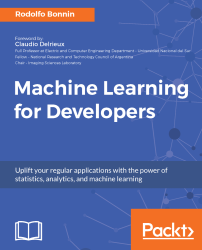•#### Machine Learning for Developers#### Overview of this book

Most of us have heard about the term Machine Learning, but surprisingly the question frequently asked by developers across the globe is, “How do I get started in Machine Learning?”. One reason could be attributed to the vastness of the subject area because people often get overwhelmed by the abstractness of ML and terms such as regression, supervised learning, probability density function, and so on. This book is a systematic guide teaching you how to implement various Machine Learning techniques and their day-to-day application and development. You will start with the very basics of data and mathematical models in easy-to-follow language that you are familiar with; you will feel at home while implementing the examples. The book will introduce you to various libraries and frameworks used in the world of Machine Learning, and then, without wasting any time, you will get to the point and implement Regression, Clustering, classification, Neural networks, and more with fun examples. As you get to grips with the techniques, you’ll learn to implement those concepts to solve real-world scenarios for ML applications such as image analysis, Natural Language processing, and anomaly detections of time series data. By the end of the book, you will have learned various ML techniques to develop more efficient and intelligent applications.
PrefaceFree Chapter
Introduction - Machine Learning and Statistical ScienceThe Learning ProcessClusteringLinear and Logistic RegressionNeural NetworksConvolutional Neural NetworksRecurrent Neural NetworksRecent Models and DevelopmentsSoftware Installation and Configuration# Understanding the problem

When solving machine learning problems, it's important to take time to analyze both the data and the possible amount of work beforehand. This preliminary step is flexible and less formal than all the subsequent ones on this list.

From the definition of machine learning, we know that our final goal is to make the computer learn or generalize a certain behavior or model from a sample set of data. So, the first thing we should do is understand the new capabilities we want to learn.

In the enterprise field, this is the time to have more practical discussions and brainstorms. The main questions we could ask ourselves during this phase could be as follows:

• What is the real problem we are trying to solve?
• What is the current information pipeline?
• How can I streamline data acquisition?
• Is the incoming data complete, or does it have gaps?